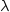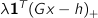# CVXGEN: Code Generation for Convex Optimization

The solver generated by CVXGEN does not explicitly handle infeasible or unbounded problems. In both cases, the solver will perform settings.max_iters steps, and terminate with work.converged == 0. This is by design.

## Handling infeasibility

If you wish to avoid infeasibility altogether, change your model so it is always feasible. One method is to replace constraints with penalty terms for constraint violation. For example, instead of an equality constraint, you could add a penalty term to the objective, with(in CVXGEN, lambda*norm_1(A*x - b)). This term is the sum of the absolute value of the constraint violations. Choosesufficiently large that the equality constraint is seldom violated. (The required value is application dependent.)

For an inequality constraint, add a penalty term to the objective(in CVXGEN, lambda*sum(pos(G*x - h))). This term is the sum of constraint violations. Again, choosesufficiently large that the inequality constraint is seldom violated.

### Using a phase I method

For total control over the behavior of your solver when no feasible point is available, consider creating an additional solver. Here, you would describe a second problem where the original constraints are replaced with constraint violation terms (as described above). This solver then provides a way to choose a point that is a compromise between feasibility and optimality in the original formulation. See Boyd and Vandenberghe,11.4, for some details and examples.

Here you might solve problems first with the phase I method, then, if the problem is feasible and bounded, use your original CVXGEN solver. Alternatively, you might use the original solver first, then upon failure to converge, switch to the alternative ‘phase I’ solver.

## Handling unboundedness

To avoid unbounded problems, add additional constraints; perhaps lower and upper bounds on some or all variables. Make these sufficiently large that they do not affect ‘normal’ solution. You could then check the tightness of these constraints to determine solution behavior.